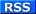Welcome Guest Search | Active Topics | Members | Log In | Register

 Euler's Equation and the Reality of Nature. Options
socratus
 Posted: Thursday, February 14, 2013 2:43:35 AMGroups: Member

Joined: 9/30/2012
Posts: 35
Points: 105
Location: Israel
Euler's Equation and the Reality of Nature.

=.

Mr. Dexter Sinister wrote:

‘ I understand Euler's Identity,

and I know what it means, and I know how to prove it,

there's nothing particularly mystical about it,

it just demonstrates that exponential, trigonometric,

and complex functions are related.

Given what we know of mathematics it shouldn't surprise

anyone that its various bits are connected.

It would be much more surprising if they weren't, that would

almost certainly mean something was badly wrong somewhere.’

Mr. Gary wrote:

Mathematics is NOT science.

Science is knowledge of the REAL world.

Mathematics is an invention of the mind.

Many aspects of mathematics have found application

in the real world, but there is no guarantee.

Any correlation must meet the ultimate test:

does it explain something about the real world?

As an electrical engineer I used the generalized

Euler's equation all the time in circuit analysis:

exp(j*theta) = cos(theta) + j*sin(theta).

So it works at that particular level in electricity.

Does it work at other levels, too?

Logic cannot prove it.

It must be determined by experiment, not by philosophizing.

====..

Thinking about theirs posts I wrote brief article:

Euler's Equation and Reality.

=.

a)

Euler's Equation as a mathematical reality.

Euler's identity is "the gold standard for mathematical beauty'.

Euler's identity is "the most famous formula in all mathematics".

‘ . . . this equation is the mathematical analogue of Leonardo

da Vinci’s Mona Lisa painting or Michelangelo’s statue of David’

‘It is God’s equation.’, ‘ It is a mathematical icon.’

. . . . etc.

b)

Euler's Equation as a physical reality.

"it is absolutely paradoxical; we cannot understand it,

and we don't know what it means, . . . . .’

‘ Euler's Equation reaches down into the very depths of existence’

‘ Is Euler's Equation about fundamental matters?’

‘It would be nice to understand﻿ Euler's Identity as a physical process

using physics.‘

‘ Is it possible to unite Euler's Identity with physics, quantum physics ?’

==.

My aim is to understand the reality of nature.

Can Euler's equation explain me something about reality?

To give the answer to this question I need to bind

Euler's equation with an object - particle.

Can it be math- point or string- particle or triangle-particle?

No, Euler's formula has quantity (pi) which says me that

the particle must be only a circle .

Now I want to understand the behavior of circle - particle and

therefore I need to use spatial relativity and quantum theories.

These two theories say me that the reason of circle – particle’s

movement is its own inner impulse (h) or (h*=h/2pi).

a)

Using its own inner impulse (h) circle - particle moves

( as a wheel) in a straight line with constant speed c = 1.

We call such particle - ‘photon’.

From Earth – gravity point of view this speed is maximally.

From Vacuum point of view this speed is minimally.

In this movement quantum of light behave as a corpuscular (no charge).

b)

Using its own inner impulse / intrinsic angular momentum

( h* = h / 2pi ) circle - particle rotates around its axis.

In such movement particle has charge, produce electric waves

( waves property of particle) and its speed ( frequency) is : c>1.

We call such particle - ‘ electron’ and its energy is: E=h*f.

In this way I (as a peasant ) can understand the reality of nature.

==.

My God, that is a naïve peasant's explanation.

It is absolutely not scientific, not professor's explanation.

Would a learned man adopt such simple and naive explanation?

Hmm, . . . problem.

In any way, even Mr. Dexter Sinister and Mr. Gary

wouldn't agree with me, I want to say them

' Thank you for emails and cooperation’

=.

Best wishes.

=.

P.S.

' They would play a greater and greater role in mathematics –

and then, with the advent of quantum mechanics in the twentieth

century, in physics and engineering and any field that deals with

cyclical phenomena such as waves that can be represented by

complex numbers. For a complex number allows you to represent

two processes such as phase and wavelenght simultaneously –

and a complex exponential allows you to map a straight line

onto a circle in a complex plane.'

/ Book: The great equations. Chapter four.

The gold standard for mathematical beauty.

Euler’s equation. Page 104. /

#

Euler's e-iPi+1=0 is an amazing equation, not in-and-of itself,

but because it sharply points to our utter ignorance of the

simplest mathematical and scientific fundamentals.

The equation means that in flat Euclidean space, e and Pi happen

to have their particular values to satisfy any equation that relates

their mathematical constructs. In curved space, e and Pi vary.

/ Rasulkhozha S. Sharafiddinov . /

===============…

"Bot"-tee-licious
 Posted: Thursday, February 14, 2013 4:00:50 AM
Groups: Member

Joined: 7/22/2012
Posts: 215
Points: 645
Location: Scotland, UK
Hi Socratus,

You might like to check this out; The decoding of the Wilton Windmill Crop Formation: http://logosmythos.net/wilton_windmill_crop_circle_may_2010.html

Cheers!
Users browsing this topic
Guest

 Forum Jump General Discussion - Open Programmed Reality - Philosophy - Digital Consciousness - Transhumanism & Singularities - Anomalies Science & Technology - AI - Cosmology - Nanotech - Quantum Mechanics - Robotics - Gaming - General You cannot post new topics in this forum. You cannot reply to topics in this forum. You cannot delete your posts in this forum. You cannot edit your posts in this forum. You cannot create polls in this forum. You cannot vote in polls in this forum.

Main Forum RSS :Universe Solved Theme Created by Jim Elvidge (Universe Solved)